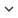ENGBook 1508
Author/s
• Fernando Pardo, Paula López and Diego Cabello
ISBN
• 978-953-307-569-3
Source
• InTech, 2011
Research Areas

# Heat Transfer for NDE: Landmine Detection

An efficient way of extracting information from infrared images regarding the presence of landmines using a 3D thermal model of the soil is presented. The approach is based on the solution of the heat equation. The process is divided in two steps. On the first one, the forward problem, the soil is subjected to a heating process and a comparison between temperatures measured at the soil surface (through IR imaging) and those obtained by simulation using the model under the assumption of homogeneous soil and mine absence is made. The differences between measured and simulated data put into evidence the presence of unexpected objects on the soil. The second step is an inverse engineering problem where the thermal model must be run for multiple soil configurations representing different types of possible targets (mine, stone, ...) and depths of burial. The nearest configuration to the measured data give us the estimated nature and location of the targets. This approach and, particularly, the inverse engineering process, makes an intensive use of the 3D thermal model that needs to be solved iteratively involving complex, coupled sets of partial differential equations. The extensive computing power required makes impractical its software implementation in personal computers. An alternative solution is the hardware implementation of the Finite Difference (FD) representation of the thermal model. In fact, different hardware implementations of FD solutions in the electromagnetics domain can be found in the literature. In previous works, we have presented an FPGA implementation of a FD Heat Equation solver which speeds the computation up by a factor of 10 compared to the purely software solution. The bottleneck of such an implementation is the access to memory and the amount of available memory, which dramatically reduces the performance of the system. However, this solution is hardware dependent an its cost is quite high. In recent years, Graphic Processing Units (GPUs) have been proved to be a valuable hardware platform to solve problems with a high degree of parallelism, Hwu et al. (2008). The total speedup of the system in a typical simulation setup by a factor 40 compared to a Core2Duo 2.8 GHz implementation in C++, using a NVIDIA GTS250 GPU, but even higher speedups could be achieved with more advanced GPUs. The chapter is outlined as follows. In Section 2 the thermal model and the detection algorithm are introduced. Section 3 addresses the architecture of the hardware implementation and its GPU projection details. In Section 4 the main results are shown and, finally, the conclusions are summarized.
Keywords: NDE, antipersonnel mines, FPGA, GPU